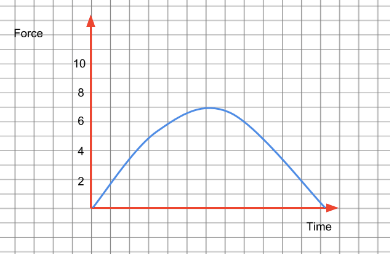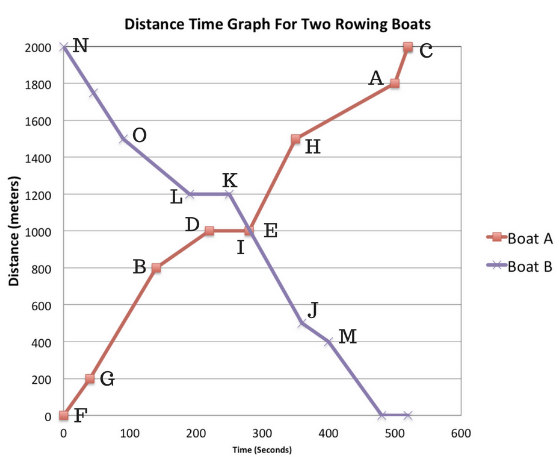### News

We’ve been hard at work developing a Rowing STEM curriculum for use with the Concept 2 rowing machines. We are currently running a pilot program at a local middle school. I started with two students three weeks ago, and now I have six students enrolled. Things are moving in the right direction! At present, we are learning how to use the machines. Concept 2 has a well-written curriculum that does incorporate some math and science activities. I wanted to develop materials to accompany this curriculum.

I present below a few activities that you are welcome to download and try in your STEM classes if you happen to have a rowing program at your school. If you do decide to use these activities, please contact me at neil@okrowing.org and let me know how your RowingSTEM activities turned out.### 1) The Force Curve (without the calculus)

This activity introduces the force curve on the Concept 2. Students spend some time learning what a good force curve looks like and why this kind of curve gets created. All of the curves in the first section are drawn on graph paper, so students can calculate the area under the curve and learn about the concept of impulse. Once they learn about the application of the force curve, they create their force curve and trace it in their graph paper. Note: if anyone knows of a way to capture the points of the force curve on a Concept 2, I would love to hear from you as I would like to improve this part of this lab.### 2) Time-Distance Graphs

This activity introduces the concept of a time-distance graph. The activity starts with a data table, and students plot these data points on a piece of graph paper. The data points correspond to two eights rowing 2000m during the data collection. Each boat starts at opposing ends of the racecourse and the distance traveled by each boat is recorded various time intervals.

Following this, students label the graph with points that correspond to coxswain commands. It would help if students are familiar with coxswain commands, but it is not necessary.### 3) Accuracy vs. Precision

This activity introduces the idea of accuracy and precision. Diagrams of dartboards demonstrate the various aspects of accuracy and precision. Students learn the difference between the two terms and draw/label on the dartboards to help with their learning. In the next part of the activity, students are instructed to use the game “Darts” on the Concept 2 rowing machine. In this part of the activity, students get to apply what they have learned and must row consistently to reach the highest score possible.### 4) Investigating Consistency and Scatter Charts

In this activity, another approach to learning about consistency and mathematical relationships, students row 500m in 100m segments. This activity introduces the idea of a trend line. The activity also introduces correlation of data. Students examine their data and decide if there is a correlation between rate and watts. Students will need access to ErgData and be able to upload their data to Concept 2 so that they can download CSV files and use Google Apps to analyze their data.

A student from the Tulsa Youth Rowing Association has also assisted me. He is getting credit for this in his high school Physics class. The lab below is a lab that Dylan Kupetsky has developed to help integrate Physics into high school Physics classes.### 5) Mass/Energy Lab

In this activity, students will learn how to calculate energy from a power versus time graph, and how to solve kinetic energy problems. Students will then use these two skills to find the virtual mass of their boat when on an ergometer.